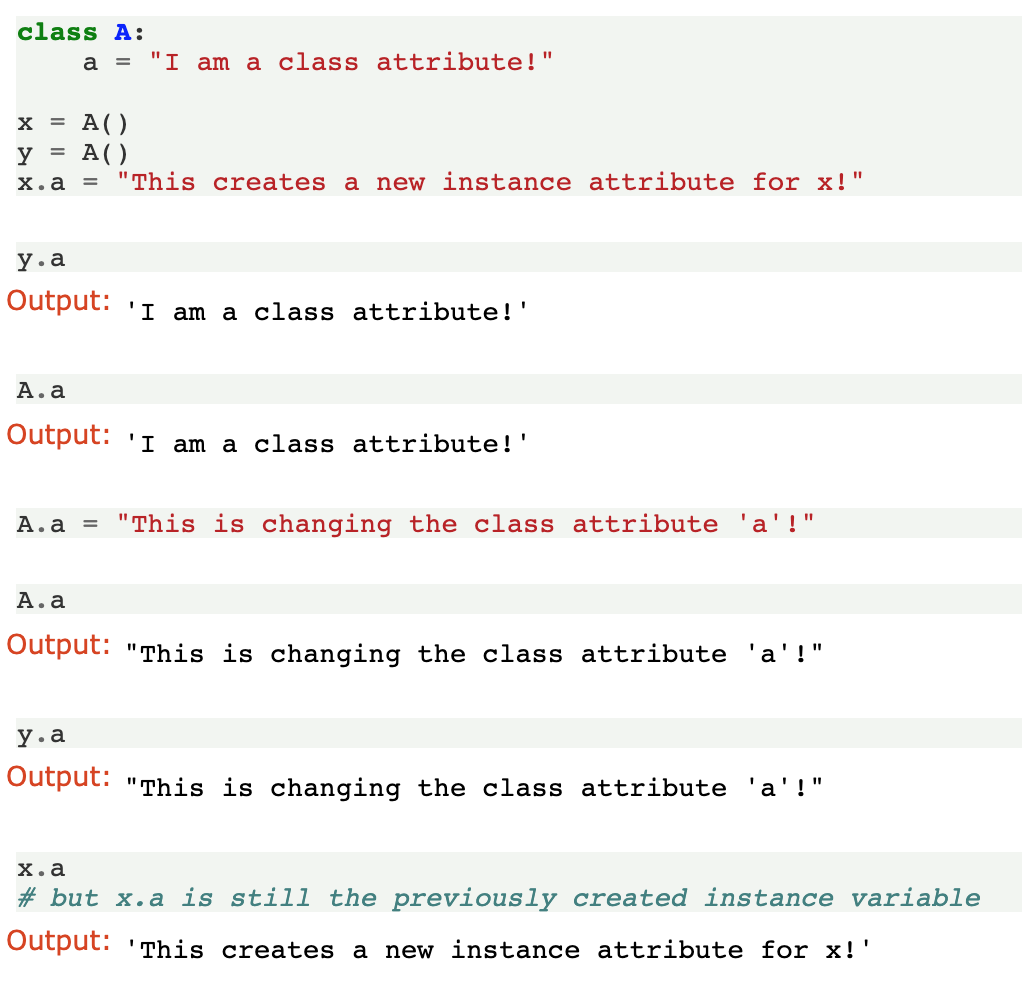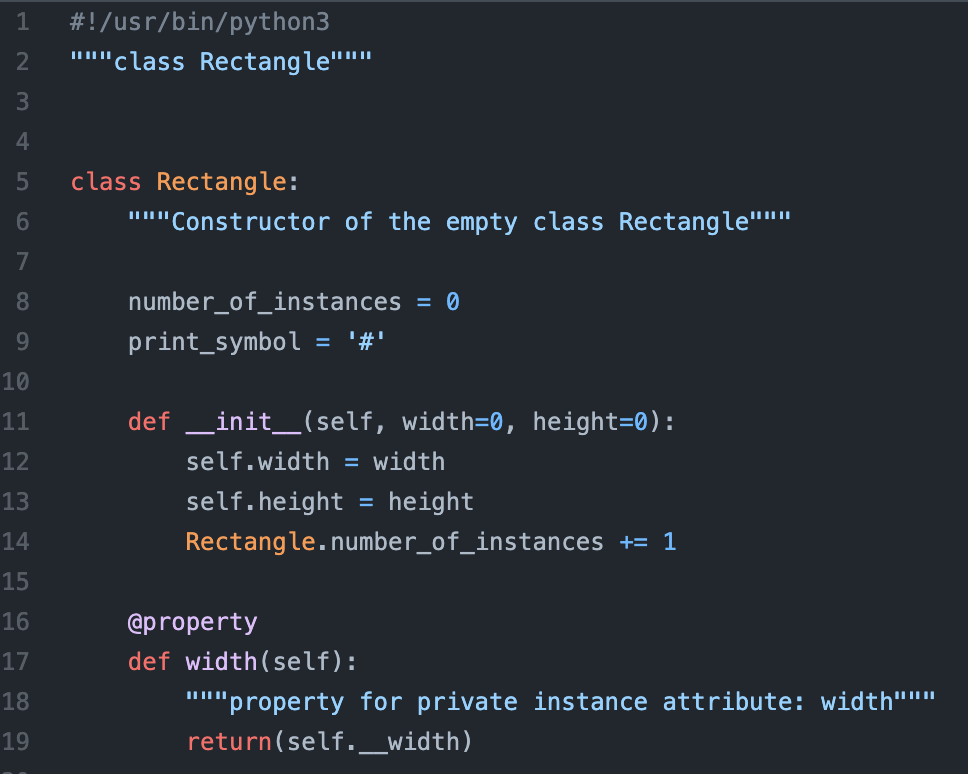Class and instance attributes

How object and class attributes workWhat can happen if we define a Human like an OOP? -> class Human

What’s a class attribute?

Class attributes are attributes which are owned by the class itself. They will be shared by all the instances of the class. Therefore they have the same value for every instance. We define class attributes outside all the methods, usually they are placed at the top, right below the class header.

What’s an instance attribute?

Instance attributes are unique for each object. That is, for two different instances, the instance attributes are usually different.we can see in the example a difference between a class attribute and an instance attribute. When we call an instance attribute, it only affects the specific instance of a class (in the example: x.a)

What are all the ways to create them and what is the Pythonic way of doing it?

In the next example, we can see the ways to create them:

• Class attribute are represented after Class Rectangle: “number_of_instances” and “print_symbol”
• Instance attribute: We create after the initializer (__init__). It is automatically called when we instantiate the class. We have to include the self parameter so that our initializer has a reference to the new object being instantiated. With this form, any Rectangle object will be able to stores it width and height
#!/usr/bin/python3
"""class Rectangle"""
class Rectangle:
"""Constructor of the empty class Rectangle"""
number_of_instances = 0
print_symbol = '#'
def __init__(self, width=0, height=0):
self.width = width
self.height = height
Rectangle.number_of_instances += 1

Now, let’s handle what is Pythonic:

A non-pythonic looks like this: The problem starts when we need to modify the code. Tha’s why the Pythonic way comes to rescue!

class Square:
"""Constructor of class Square"""
def __init__(self, size=0):
if not isinstance(size, int):
raise TypeError("size must be an integer")

if size < 0:
raise ValueError("size must be >= 0")

self.__size = size * size
def area(self):
return self.__sizet__width()

In the Pythonic way, we see that we use “@property” “setter” and “getter” methods:

class Square:
"""Constructor of class Square"""
def __init__(self, size=0):
self.size = size
@property
def size(self):
return self.__size
@size.setter
def size(self, value):
if not isinstance(value, int):
raise TypeError("size must be an integer")
if value < 0:
raise ValueError("size must be >= 0")
self.__size = value
def area(self):
return self.__size * self.__size

The Pythonic method allow us to set or attach code to the self.size attribute and the new “value” will be the next size of the instance attribute

What are the differences between class and instance attributes?

The big difference between them:

• A class attributes are shared, so we can say that they can be accessed by all instances of that class. In the next example, we can see that “number_of_instances” and “print_symbol” will be shared to all Rectangles instance of the “class Rectangle”. And the value is the same. We can see that in the next example we use to have a counter of instances of the “class Rectangle” and also define a symbol that we will print the square. These class attributes values will be the same for all instances of the classExample of class Rectangle Class which has class and instance attributes
• Instance attributes are owned by each individual instance of the class. We can have a good example in the next image: We can see that each object (Dog1, Dog2, Dog3) have different instance attributes (Breed, Size, Colour and Age values are different for each one)

What are the advantages and drawbacks of each of them?

They have different usabilities as we check in the before point:

Advantages and drawbacks of Class Attribute:

• They store a variable that are shared between all object. We can use it to store data relevant like number of instance of the class or another
• If we need the same variable with the same value for all the instances of the class, we can choose a Class Attribute
• One disadvantage is when an object name attribute has the same name as the class variable. They will hide the class variable

Advantages and drawbacks of Instance Attribute:

• We can create a instance attribute and it will have different values for each object of the class, so it will easy to handle it
• We can use the pythonic way to access to the properties, getter and setter methods, so we can set an attribute of a specific instance easily
• You can not update all the instance attribute at the same time like the Class attribute and it is because it is different for each object. They have different uses

How does Python deal with the object and class attributes using the __dict__ ?

A special attribute of every module is __dict__. This is the dictionary containing the module’s symbol table.

object.__dict__

So, thanks to this module, each instance is stored in a dictonary. Let’s check examples:

How we can use it?

class Robot:
pass

x = Robot()
y = Robot()

x.name = "Marvin"
x.build_year = "1979"

y.name = "Caliban"
y.build_year = "1993"

and how we can use with object attributes, let’s check the ouput:

x.__dict__
# Output:
{'name': 'Marvin', 'build_year': '1979'}
y.__dict__
# Output:
{'name': 'Caliban', 'build_year': '1993'}

In the next example, we will check how it works with class attribute:

class MyClass(object):
class_var = 1

def __init__(self, i_var):
self.i_var = i_var

foo = MyClass(2)
bar = MyClass(3)

print MyClass.__dict__
print foo.__dict__
print bar.__dict__

and let’s check the ouput:

{'__module__': '__main__', 'class_var': 1, '__dict__': <attribute '__dict__' of 'MyClass' objects>, '__weakref__': <attribute '__weakref__' of 'MyClass' objects>, '__doc__': None, '__init__': <function __init__ at 0x0000000004E55CF8>}
{'i_var': 2}
{'i_var': 3}